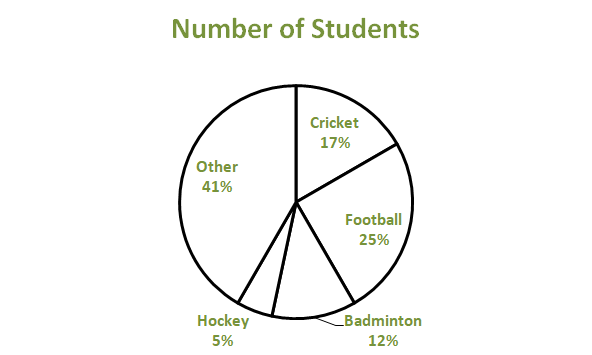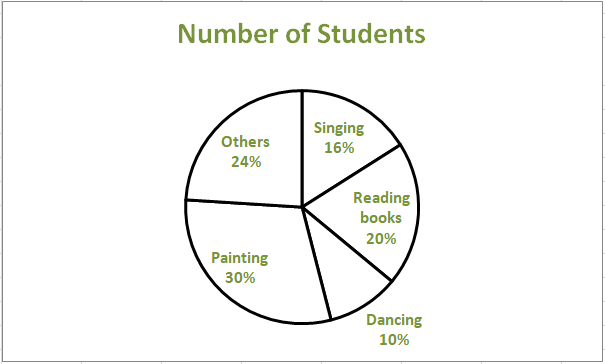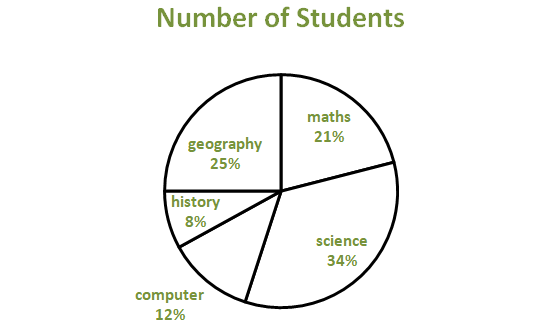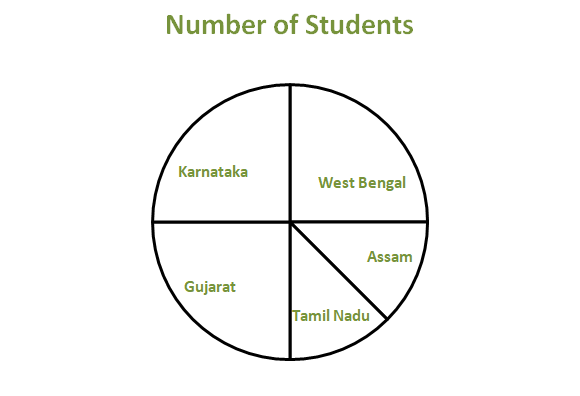Related Articles
Pie Chart – Class 8
• Last Updated : 19 Jan, 2021

A Circle Graph or a Pie Chart, in Data handling, is one type of pictorial representation of a given set of data, which is used to illustrate numerical problems by dividing a circular statistical graphic into slices or sectors. Here, the term “pie” corresponds to the word whole, while the term “sectors” or “slices” corresponds to parts of the whole. Pie charts are basically used to find the composition of something. The best real-life example of a circle graph or a pie chart is Pizza. Let us see another example for pie charts.

### Example

In a class of 60 students, a survey was done to collect each student’s favorite sports. The collected data is listed as:

If we try to represent the above data using a pie chart, the resultant pictorial representation will be as follows:### Formula

The total value or percentage of the pie is 100% always. Here it contains different sectors and segments in which each sector or segment of the chart corresponds to a certain portion of the net or total percentage (or data). The total or sum of all the data can be summed up to 360 degrees.

How to calculate the degrees of each slice in a pie chart from the percentage?

To work out with the given percentage in a pie chart, we need to follow the following steps:

• Categorize the data.
• Then calculate the total.
• Now we need to divide the categories.
• After which, convert it into percentages.
• And finally, calculate the degrees.

The formula for a pie chart can be summed up as:

(Given Data / Total Value of Data) x 360

How to calculate the percentage of each sector from degrees in a pie chart?

To work out with degrees in a pie chart, we need to follow the following steps:

• At first, we need to measure every slice of the chart.
• Then we need to divide it by 360 degrees.
• And finally, multiply the obtained result by 100.

How to calculate the total number of sectors on a pie chart?

To calculate the total number of slices or sectors on a pie chart, we need to multiply the sector’s percentage by the total value of the data and finally divide the result by 100.

### Creating a Pie Chart

We will learn how to create a pie chart step by step with the help of an example.

Example:

A teacher surveyed a group of students to see what is the favorite hobby of each student. The data collected is listed as follows:

Now we will see how to construct a pie chart step by step.

Step 1: The first step requires us to jot down the available data into tabular form as follows:

Step 2: The next task is to calculate the sum of all the given data.

Here, the Sum of All Data = (16 + 20 + 10 + 30 + 24) =100

Step 3: Now, the next task is to calculate the percentage of each sector. We need to divide each sector value by the sum or total and then multiply it by 100.

Step 4: Next step is to calculate the degrees corresponding to each slice. The values can be calculated as:

central angle of each component = (Given Data / Total Value of Data) x 360

Hence, The values are as follows:

Step 5: Now, with a protractor’s help, we will measure each angle from a single point or central point and draw the circle’s sectors. The resultant pie chart will be:### Solving Problems

We will now see how to solve mathematical problems using a pie chart.

Question 1: The given pie chart shows the subject of interest of each student in a class.Answer the following question concerning the given pie diagram.

1. If 30 student’s subjects of interest are history, how many total students were surveyed?
2. Which subject is liked the most?
3. Which subject is disliked the most?

Solution:

1. According to the given question, 8% of the total number of students is 30.
i.e. (8/100) x Total = 30
Therefore, Total = 30 x (100/8) = 375
Hence 375 students were surveyed.

2. According to the given pie chart, science is liked the most.

3. According to the given pie chart, history is disliked the most.

Question 2: For a science camp, students from different states have enrolled. Construct a pie chart for the given table:

Solution:

Step 1: The first step requires us to jot down the available data into tabular form as follows:

Step 2: The next task is to calculate the sum of all the given data.

Here, the Sum of All Data = (10 + 5 + 5 + 10 + 10) = 40

Step 3: Now, the next task is to calculate the percentage of each sector. We need to divide each sector value by the sum or total and then multiply it by 100.

Step 4: Next step is to calculate the degrees corresponding to each slice. The values can be calculated as:

Step 5: Now, with a protractor’s help, we will measure each angle from a single point or central point and draw the circle’s sectors. The resultant pie chart will be: